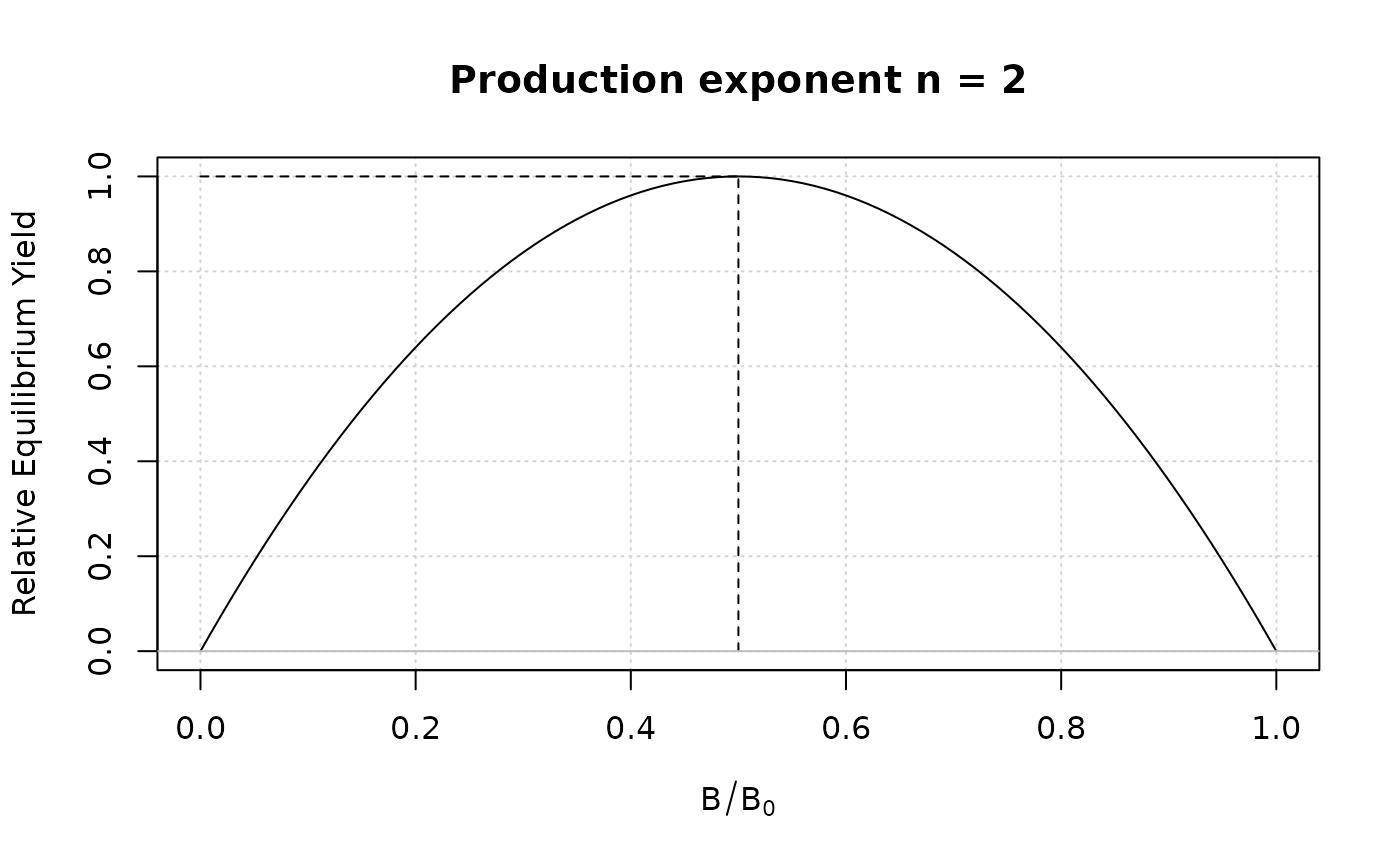For surplus production models, this function returns the production exponent n corresponding to BMSY/K (Fletcher 1978).

SP_production(depletion, figure = TRUE)

## Arguments

depletion

The hypothesized depletion that produces MSY.

figure

Local, plots figure of production function as a function of depletion (B/K)

## Value

The production function exponent n (numeric).

## Note

May be useful for parameterizing n in SP and SP_SS.

SP_production(0.5)